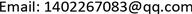1. 前言

2. 理论分析与假设

3. 实证检验3.1. 建立模型

D = β 0 + β 1 x 1 + β 2 x 2 + β 3 x 3 + β 4 x 4 + μ

Model parameter lis

−811.14154.71−5.240.0000
1430.26425.053.360.0002
1.160.186.530.0000
−0.170.04−4.170.0000

3.2. 异方差检验

H 0 : 对 全 体   i   有 σ i 2 = σ 2

H 1 : σ i 2 不 全 等 于 σ 2

μ ^ 2 = δ 0 + δ 1 y ^ + δ 2 y ^ 2 + v

3.3. 异方差的修正

β ^ FGLS = ( X ′ Ω ^ − 1 X ) X ′ Ω ^ − 1 y

FGLS estimation results of the mode

x 1−791.55164.29−4.820.0000
x 21526.33425.873.580.0001
x 30.930.165.900.0000
x 4−0.150.04−3.850.0001

D = 7510863 − 791.55 x 1 + 1526.33 x 2 + 0.93 x 3 − 0.15 x 4

4. 结论